## 4.1.8 Long Term Predictor lag determination

06.203GPPHalf rate speech transcodingTS

Figure 3 illustrates that the long term lag optimization looks just like a codebook search where the codebook is defined by the long term filter state and the specific vector in the codebook is pointed to by the long term predictor lag, L. The input p(n) is the weighted input speech for the subframe minus the zero input response of just the H(z) filter.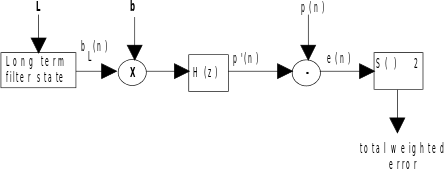Figure 3: Long term predictor lag search

The GSM half rate speech encoder uses a combination of open loop and closed loop techniques in choosing the long term predictor lag. First an open loop search is conducted to determine "candidate" lags at each subframe. Then at most, two best candidate lags at each subframe are selected, with each serving as an anchor point for constructing an open loop frame lag trajectory, subject to a maximum delta coding constraint. The frame lag trajectory which minimizes the open loop LTP spectrally weighted error energy for the frame is then chosen. The open loop LTP prediction gains corresponding to the winning trajectory are used to select the voicing mode 1, 2 or 3. If MODE¹0, the closed loop lag evaluation is initiated. The winning trajectory has associated with it a list of lags to be searched closed loop at each subframe.

It is possible to allow L to take on fractional values, thus increasing the resolution, and in turn the performance, of the adaptive codebook. Table 3 shows the allowable lags.

Table 3: Allowable lags

 Range Resolution Number of lags in range 21 to 22 2/3 1/3 6 23 to 34 5/6 1/6 72 35 to 49 2/3 1/3 45 50 to 89 1/2 1/2 80 90 to 142 1 53

The resolution of the long term filter state may be increased by upsampling and filtering the state. In this implementation, a non-causal, zero-phase Finite Impulse Response (FIR) filter is used. Where needed, the future samples for the non-causal filtering operation are replaced by the output of the predictor.

#### 4.1.8.1 Open loop long term search initialization

An open-loop lag search is done to narrow the range of lags over which a closed-loop search will eventually be performed.

The first steps of the open-loop subframe lag search are as follows:

 STEP 1 Initialize the subframe counterm=1 STEP 2 The autocorrelation sequence of y(n) the input speech, s(n), filtered by W, is calculated for all allowable integer lags, and for a few integer lags below and above the lower and upper limits for the current subframe.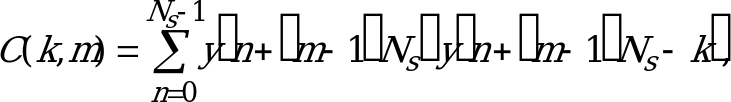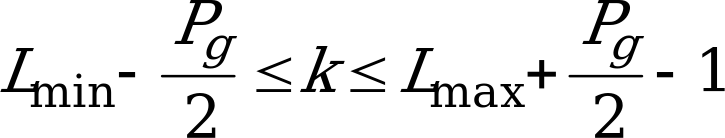(42)where Lmin = 21 and Lmax = 142.The value Pg is the order of one phase of the interpolating FIR filter used to interpolate the correlations. The energy of y(n) for the subframe is computed: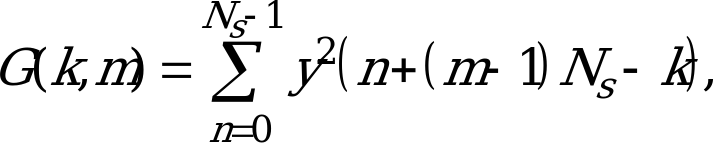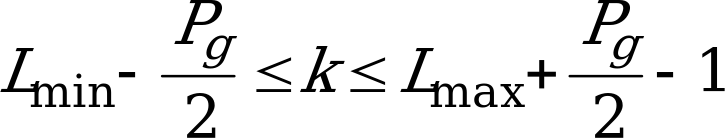(43) STEP 3 These arrays, C(k,m) and G(k,m), are searched for the integer lag which maximizes C2(k,m)/G(k,m) where C(k,m) and G(k,m) need to be greater than 0. STEP 4 If a valid maximum is found in step 3, the values for the lag, C, and G corresponding to the maximum are retained in the arrays as Lpeak(0,m), Cpeak(0,m), and Gpeak(0,m).Otherwise, Lpeak(0,m) = Lmin (44) Cpeak(0,m) = 0 (45) Gpeak(0,m) = 1 (46) STEP 5 m=m+1 STEP 6 If m £ 4, go to step 2 STEP 7 Calculate the open loop frame LTP prediction gain: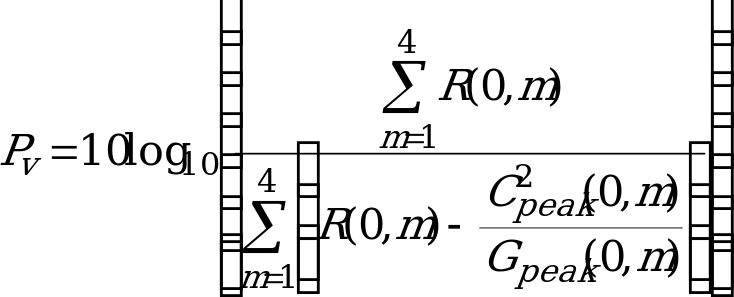(47)where: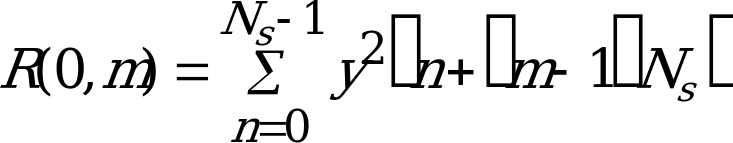, (48) STEP 8 Determine if the voicing mode is unvoiced:If Pv < 1,7 then MODE=0, the long term predictor is disabled and the open loop and closed loop lag searches are aborted. In this case, proceed to subclause 4.1.10.

#### 4.1.8.2 Open loop lag search

When MODE ¹ 0, the lag search processing is continued. The next part of the search finds the allowable lag (see table 3) which maximizes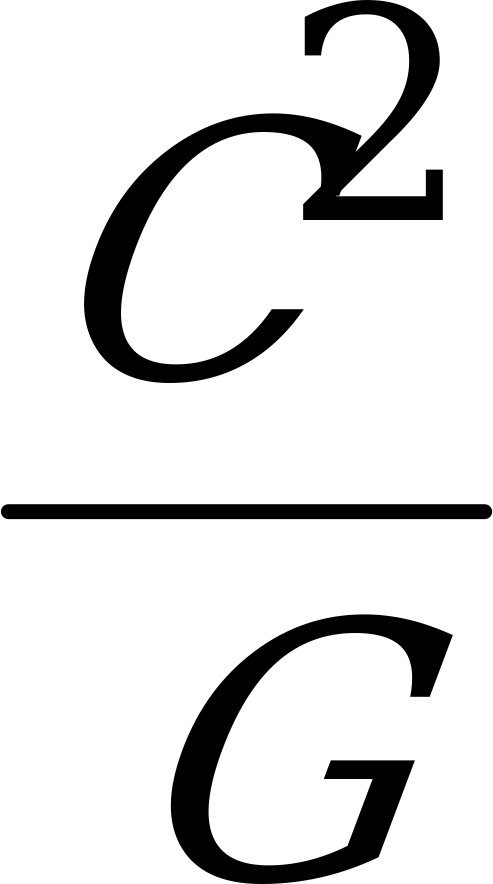in the vicinity of the best open-loop integer resolution lag, Lpeak(0,m), for values of C > 0.

 STEP 1 Initialize the subframe counterm=1 STEP 2 Initialize the peak indexLp,m = 0 STEP 3 Using interpolated versions of the C and G arrays, allowable lag values k’ in the range:Lpeak(0,m) – 1 < k’ < Lpeak(0,m) + 1 (49)are searched for a k which maximizes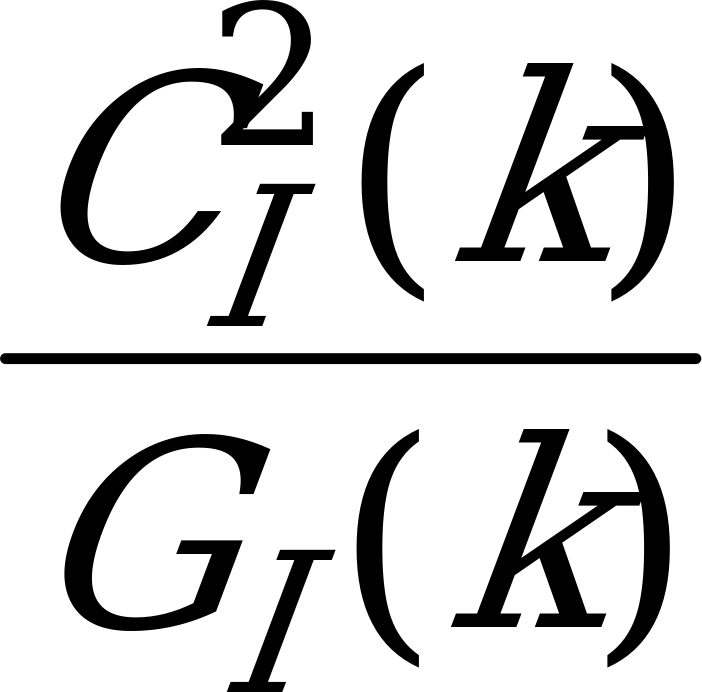(50)where: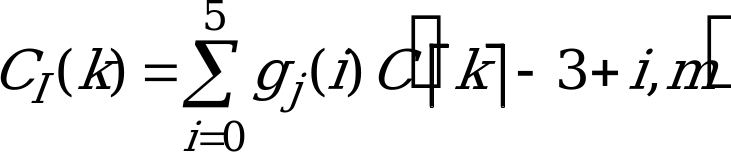(51)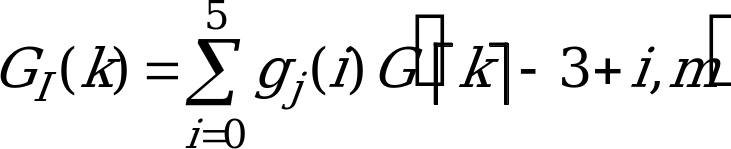(52)and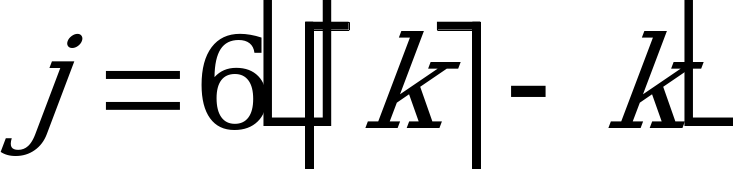(53)The coefficients of the interpolating filter are gj(i) for 0 £ i £ 5.Only CI(k) > 0 and GI(k) > 0 values are considered. If no positive correlation is found, then sethnw,m = 0, Lpeak(1,m)=Lmin, and go to Step 22.Otherwise, store the information related to the valid best allowable lag k.Lp,m=Lp,m+1 (54)Lpeak(Lp,m,m)=k (55)Cpeak(Lp,m,m)=CI(k) (56)Gpeak(Lp,m,m)=GI(k) (57)The next part of the search evaluates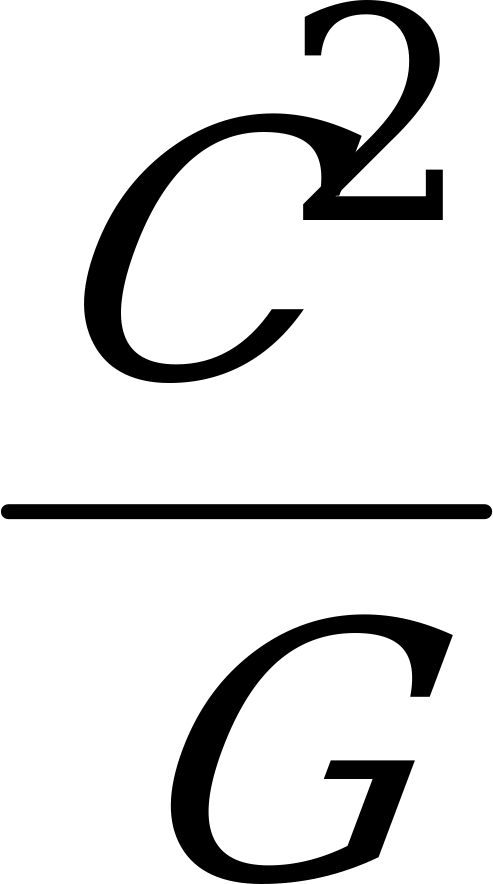, for C > 0 and G > 0, at the submultiples of the lag Lpeak(Lp,m,m) to find candidate peaks. STEP 4 Initialize the divisorJ = 2 STEP 5 Find nearest integer lag corresponding to submultiple of maximum peakk1 = round[Lpeak(1,m)/J] (58) STEP 6 Determine if submultiple is within allowable lag rangeIf k1 < LminGo to step 12 STEP 7 Find value of k’ where C2(k’,m)/G(k’,m) is a maximum for:max(Lmin,k1‑3) £ k’£ min(Lmax,k1+3) (59)If either C(k’,m) £ 0 or G(k’,m) £ 0 go to step 11. STEP 8 Determine if maximum in step 7 is a peakIf: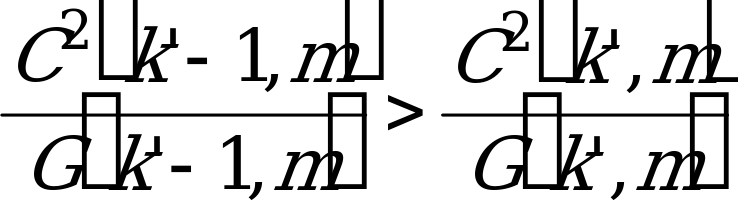(60)Go to step 11If: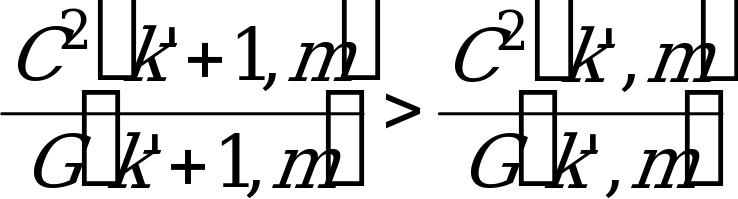(61)Go to step 11 STEP 9 A peak has been found at an integer lag, k’. Using interpolated versions of the C and G arrays, allowable lag values within + 1 (exclusive) of k’ are searched.Find k where: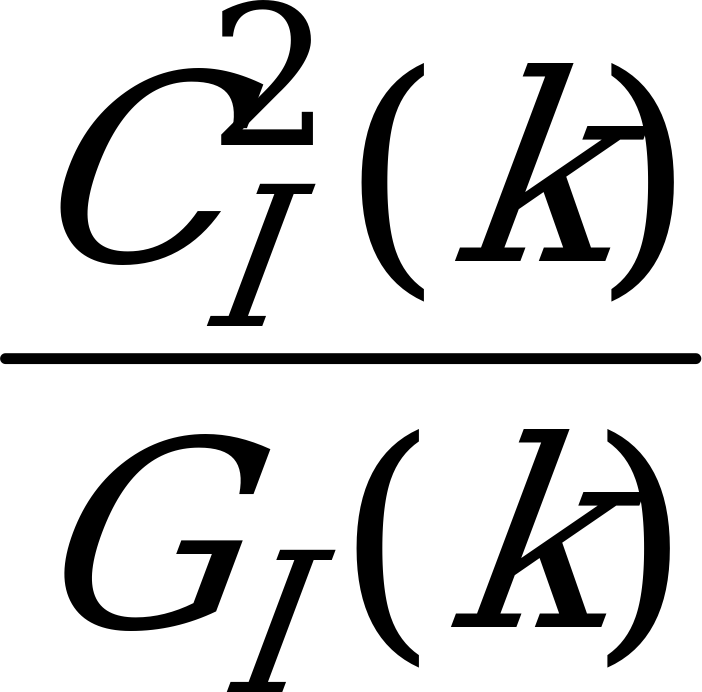(62)is a maximum, where: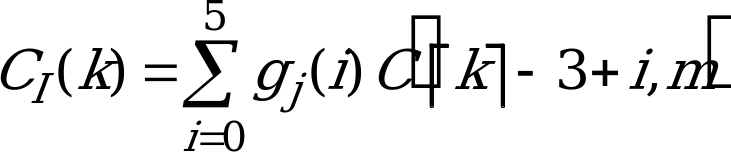(63)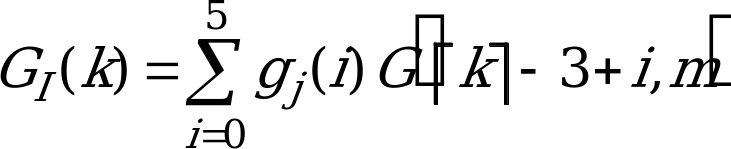(64)where: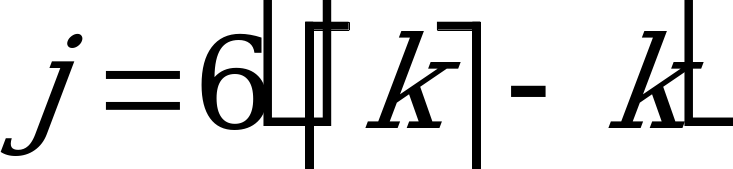(65)and k’‑1 < k < k’+1 (66)Only CI(k) > 0 and GI(k) > 0 are considered. STEP 10 If the prediction gain exceeds a threshold, the corresponding lag, CI, and GI are stored in the Lpeak(), Cpeak(), and Gpeak() arrays; otherwise, these values are not stored.If: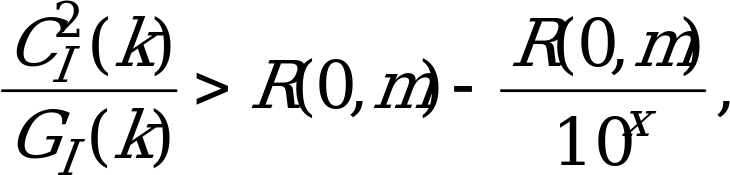where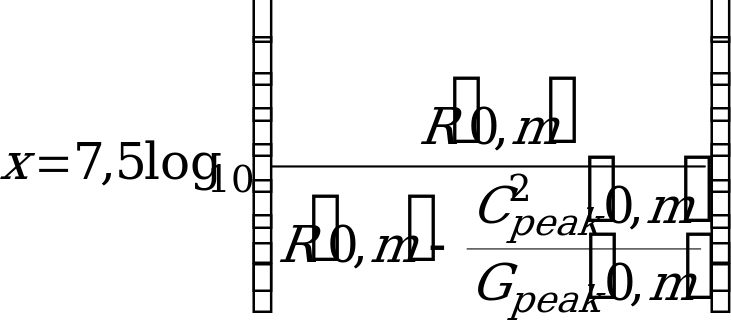(67) thenLp,m=Lp,m+1 (68)Lpeak(Lp,m,m)=k (69)Cpeak(Lp,m,m)=CI(k) (70)Gpeak(Lp,m,m)=GI(k) (71) STEP 11 Increment divisor and check the next submultipleJ=J+1Go to step 5 STEP 12 A full-resolution search (1/6 sample resolution) is done for a peak within 1 integer lag (exclusive) of the shortest lag.Find k such that: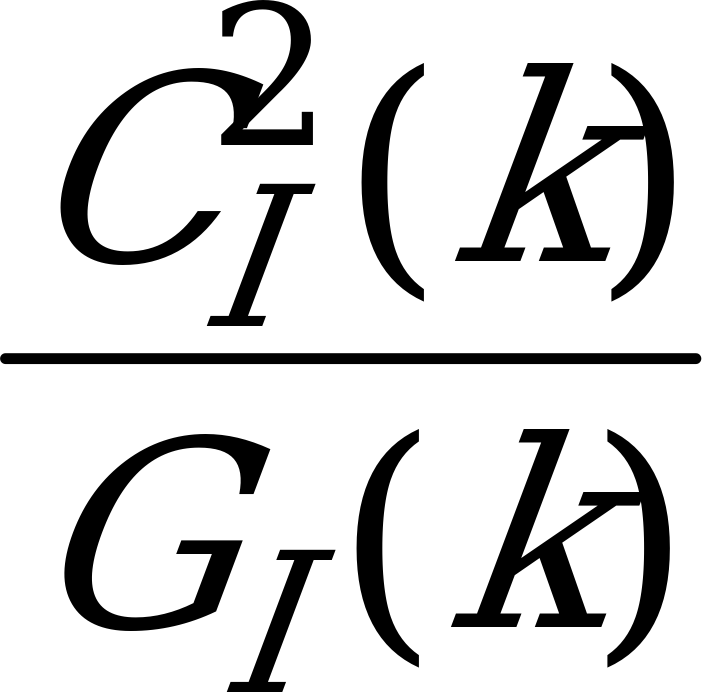(72)is a maximum, where: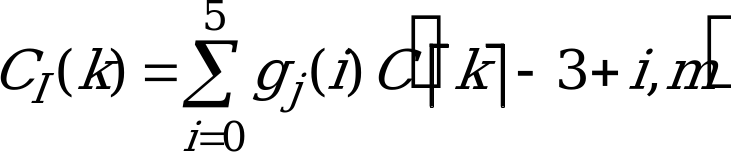(73)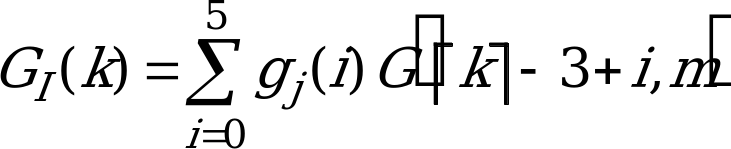(74)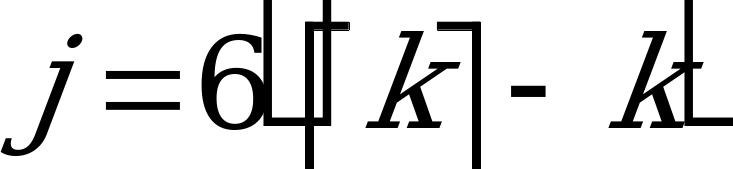(75)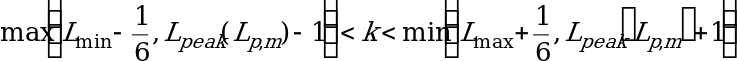(76)The fractional lag corresponding to the maximum is referred to as Lpitch,m. This lag is used by the harmonic noise weighting function C(z) at subframe m. Then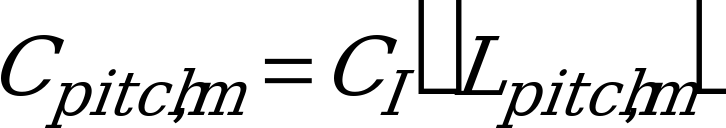(77)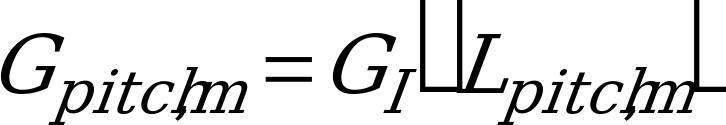(78) STEP 13 The harmonic noise weighting coefficient for subframe m is calculated in this step (see subclause 4.1.9).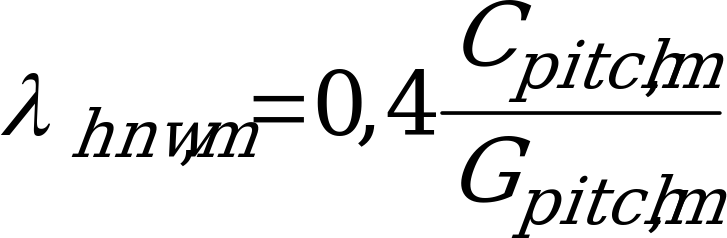(79)Once all the correlation peaks associated with submultiples of the Lpeak(1,m) have been examined, the correlation peaks associated with multiples of Lpitch,m are examined. STEP 14 Initialize the multiplierJ = 2 STEP 15 Find nearest integer lag corresponding to a multiple of the fundamental lag.k1 = round [Lpitch,m*J] (80) STEP 16 Determine if multiple is within allowable lag range.If:k1> LmaxGo to step 22 STEP 17 Find value of k’ where C2(k’,m)/G(k’,m) is a maximum for:max(Lmin,k1‑3) £ k ‘£ min(Lmax,k1+3) (81)If either C(k’,m) £ 0 or G(k’,m) £ 0 go to step 21. STEP 18 Determine if maximum in step 17 is a peak.If: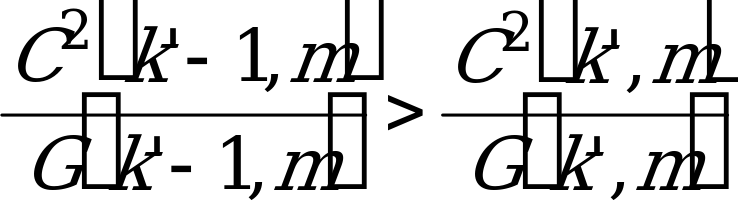(82)Go to step 21If: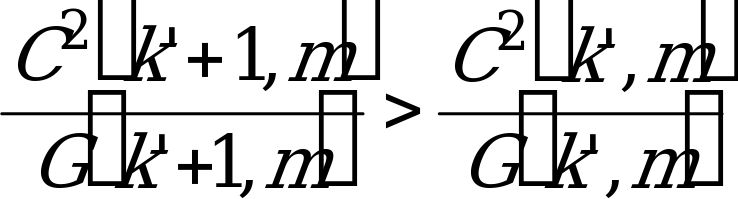(83)Go to step 21 STEP 19 A peak has been found at an integer lag, k’. Using interpolated versions of the C and G arrays, allowable lag values within + 1 (exclusive) of k’ are searched.Find k where: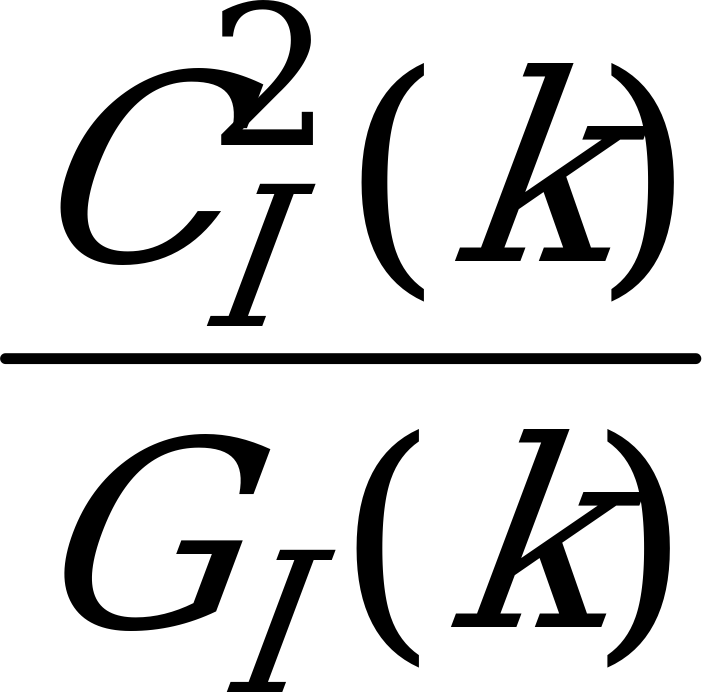(84)is a maximum, where: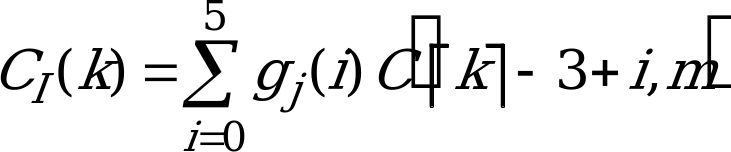(85)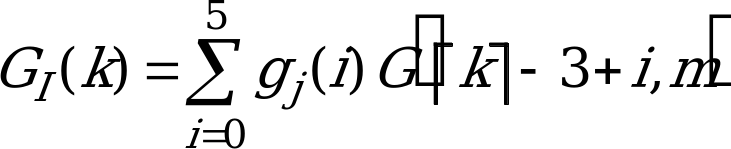(86)where: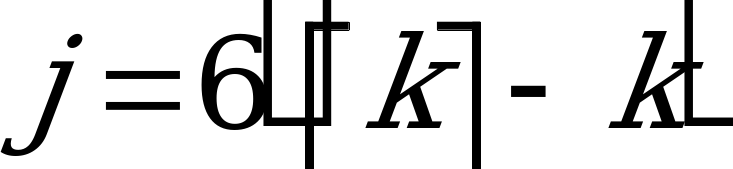(87)and k’‑1 < k < k’+1 (88)Only CI(k) > 0 and GI(k) > 0 are considered. STEP 20 If the prediction gain exceeds a threshold, the corresponding lag, CI, and GI are stored.If: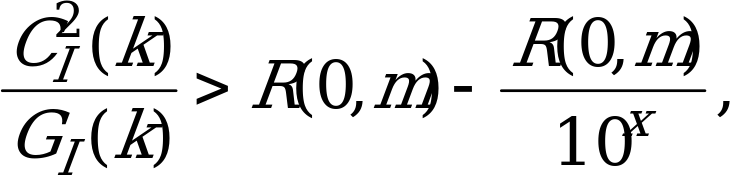where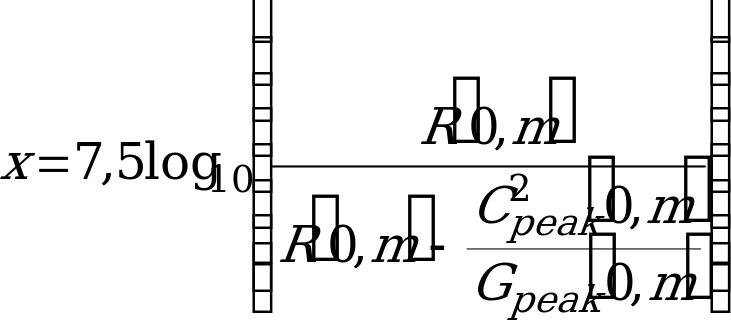(89)thenLp,m=Lp,m+1 (90)Lpeak(Lp,m)=k (91)Cpeak(Lp,m)=CI(k) (92)Gpeak(Lp,m)=GI(k) (93) STEP 21 Increment multiplier and check the next multiple.J=J+1Go to step 15 STEP 22 Increment subframe pointer and repeat for all subframes.m=m+1If: m£4Go to step 2.Otherwise, the list of correlation peaks and the harmonic noise weighting filter parameters for each subframe have been found.

#### 4.1.8.3 Frame lag trajectory search (Mode ¹) 0)

The frame lag trajectory search uses the list of potential lag values to determine the one lag value for each subframe which minimizes the open loop prediction error energy for the frame subject to the constraints of the delta lag coding employed for subframes 2, 3 and 4. Several candidate lag trajectories are determined. The trajectory which minimizes the open loop prediction error energy for the frame is chosen.

In subclause 4.1.8.2, the open loop lag search found a list of lags, Lpeak(i,m), corresponding to the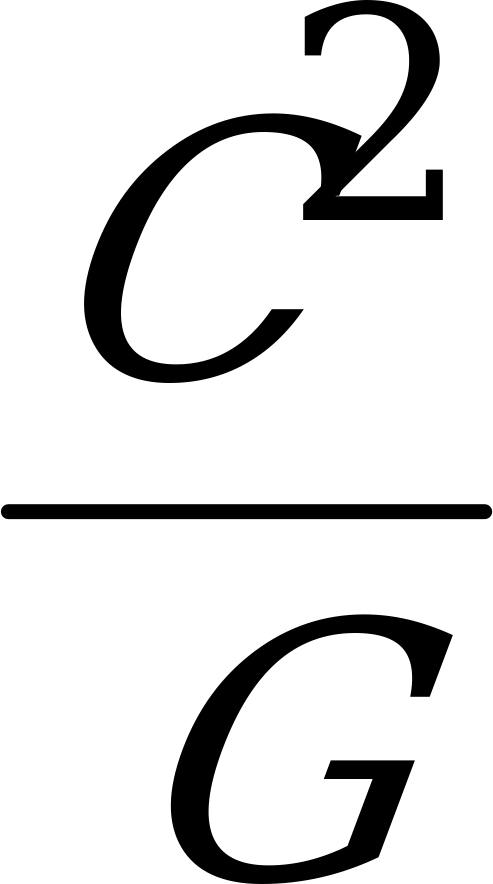peaks, for each subframe. Each trajectory evaluation begins with one of the subframes and selects a lag corresponding to a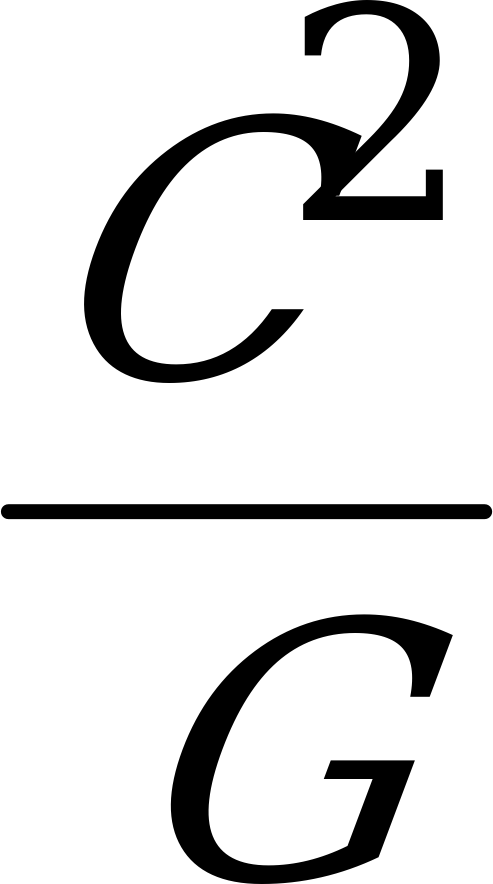peak for that subframe as the anchor for that candidate trajectory.
A maximum of 2 trajectories are anchored per subframe. From the anchor lag, the trajectory is extended forward and backward to the adjacent subframes in the frame subject to the lag differential coding constraints. The lag for each subframe on the trajectory is chosen to minimize the open loop frame prediction error energy. The trajectory search is described below.

The steps involved in the frame lag trajectory evaluation and selection are:

 STEP 1 Set m, the pointer to the selected subframe, equal to 1. STEP 2 Choose the lag at the selected subframe, m, to be an anchor lag for the frame lag trajectory; i.e., the frame lag trajectory being evaluated needs to pass through that lag. The lag which is chosen, corresponds to the highest peak in the list of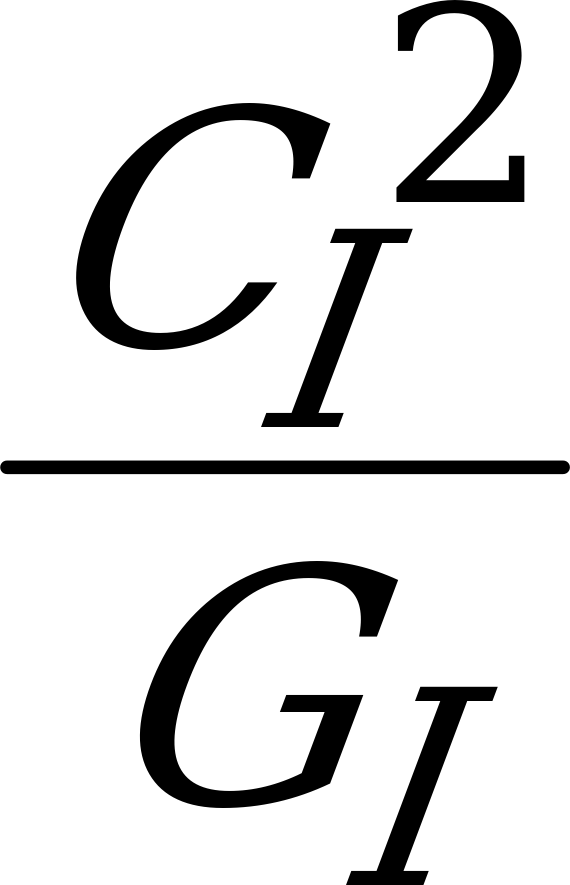peaks at subframe m, which has not been crossed by a trajectory evaluated previously. If no peaks qualify, no peaks are left, or two trajectories have already been anchored and evaluated at subframe m, go to step 7. Otherwise, compute the open loop subframe weighted error energy corresponding to the chosen lag, and store the result in the frame weighted error accumulator corresponding to the trajectory currently being evaluated. STEP 3 If m < 4, begin the forward search: STEP 3a Define the current subframe to be m+1. STEP 3b Define the forward search range as ‑7 to +6 levels relative to the current subframe’s lag level. STEP 3c Check that the lower bound does not point to a level below the lowest allowable lag level, clipping if necessary. Similarly, check that the upper bound does not point past the highest allowable lag level; clip if necessary. STEP 3d Find the lag within the range which maximizes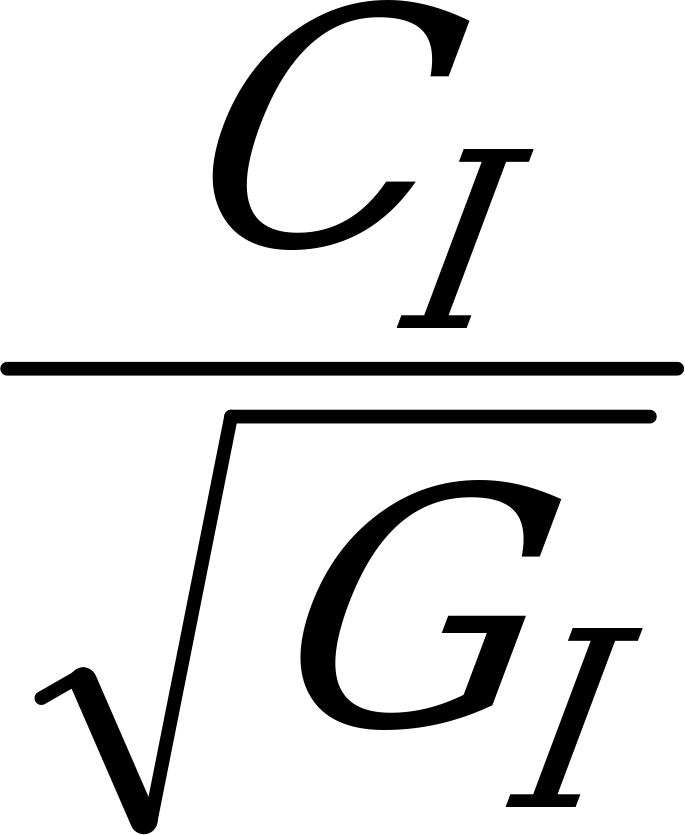.

NOTE: Negative values of CI are allowed. Compute the open loop subframe weighted error energy corresponding to that lag at the current subframe, and add the result to the frame weighted error accumulator corresponding to the trajectory being evaluated.

 STEP 3e If the current subframe < 4, increment the pointer to the current subframe, and go to step 3b. STEP 4 If m > 1, initiate the backward search: STEP 4a Define the current subframe to be m‑1. STEP 4b Define the backward search range as ‑6 to +7 levels relative to the current subframe’s lag level. STEP 4c Check that the lower bound does not point to a level below the lowest allowable lag level, clipping if necessary. Similarly, check that the upper bound does not point past the highest allowable lag level; clip if necessary. STEP 4d Find lag within the range which maximizes..

NOTE: Negative values of CI are allowed. Compute the open loop subframe weighted error energy corresponding to that lag at the current subframe, and add the result to the frame weighted error accumulator corresponding to the trajectory being evaluated.

 STEP 4e If the current subframe index is > 1, decrement the pointer to the current subframe, and go to step 4a. STEP 5 Store the lags defining the frame lag trajectory derived and the open loop LTP frame weighted error energy which this trajectory yields. Increment the counter of evaluated frame lag trajectories. STEP 6 Go to step 2. STEP 7 If m < 4, increment m and go to step 2. STEP 8 Choose, from the set of constructed frame lag trajectories, a lag trajectory which yields the lowest LTP weighted error energy for the frame, as the selected frame lag trajectory.

#### 4.1.8.4 Voicing mode selection

The frame lag trajectory is specified by a vector K={k1,k2,k3,k4}, where km is the open loop LTP lag at the m-th subframe. Define the interpolated correlation of the input spectrally weighted speech y(n) at the m-th subframe, specified by lag km, as CI(km,m) and the interpolated energy of y(n), delayed by km samples relative to the m-th subframe, as GI(km,m).

The open loop LTP prediction gain in dB at the m-th subframe is: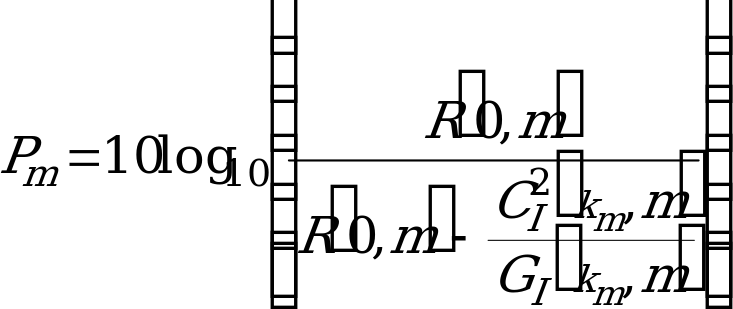(94)

The open loop frame LTP prediction gain, is given by: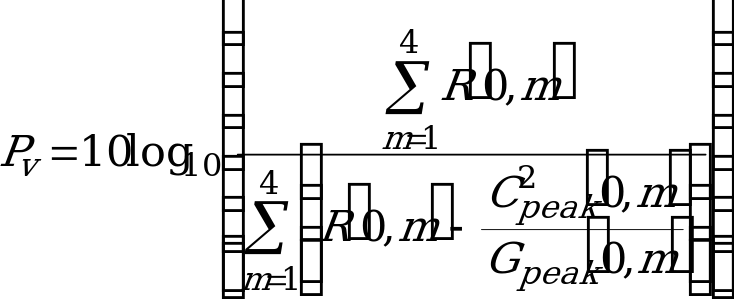(95)

The rules for mode selection are specified as follows:

MODE=0 if Pv < 1,7 (96)

MODE=1 if Pv ³ 1,7 and Pm < 3,5 for any m (97)

MODE=2 if Pm ³ 3,5 for all m and Pm < 7 for any m (98)

MODE=3 if Pm ³ 7,0 for all m (99)

#### 4.1.8.5 Closed loop lag search

From the selected frame lag trajectory, develop a list of lags to be searched closed loop. At each subframe, three allowable lag levels centred around the subframe lag, specified by the selected frame lag trajectory, will be searched. If the lag points to the lowest or the highest level in the table of quantized lag values, only two closed loop lag evaluations will be done at that subframe, with the lag outside the quantizer range being eliminated from consideration. The closed loop evaluation of the subframe lags is not performed if MODE=0. What follows is a description of the construction of the output of the long term predictor (adaptive codebook) for a given, possibly fractional lag, L. Defining:

Lmax maximum possible value for long term lag L.

r(n) long term filter state; n < 0 (history of the excitation signal).

rL(n) long term filter state with adaptive codebook output for L appended.

bL(n) output of long term filter state (adaptive codebook) for lag L.

Pf order of one phase of the interpolating FIR filter (Pf = 10 except for the special case when j = 0, see below).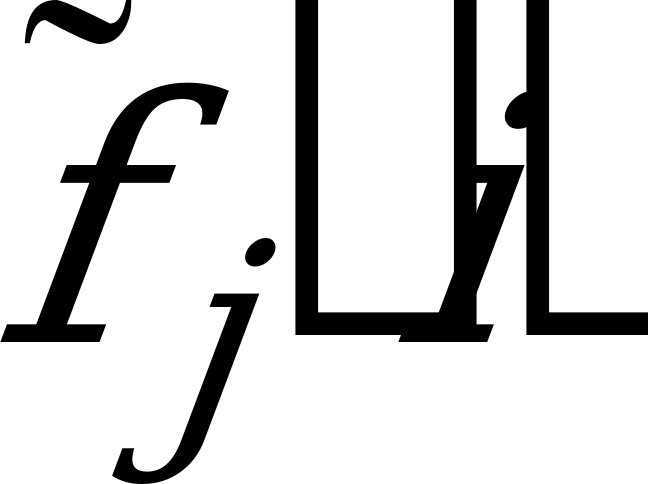coefficients of jth phase of interpolating FIR filter, i=0 to i=Pf ‑1.

Ns number of samples per subframe (Ns = 40).

The sequence rL(n) is defined as: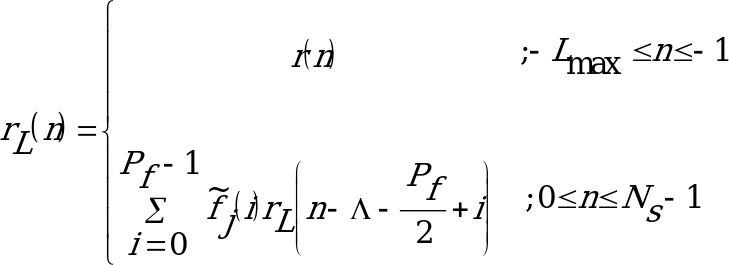(100)

where;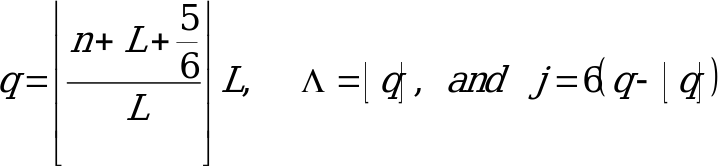The portion of the sequence rL(n) from n=0 to n=Ns‑1 shall be calculated in order from 0 to Ns‑1, so that the necessary terms in the sum will be available. The 0th phase of the interpolating filter,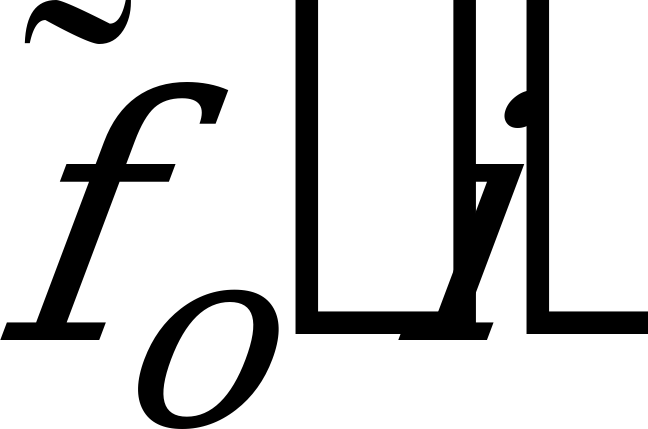, is a special case and has only one non-zero tap, so that if q is an integer, the summation reduces to the single term, rL(n-q).

The output of the codebook for lag L is just the last Ns samples in the sequence rL(n).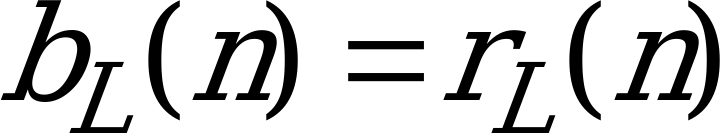;0 £ n £ Ns‑1 (101)

The closed loop search minimizes the weighted error by maximizing the term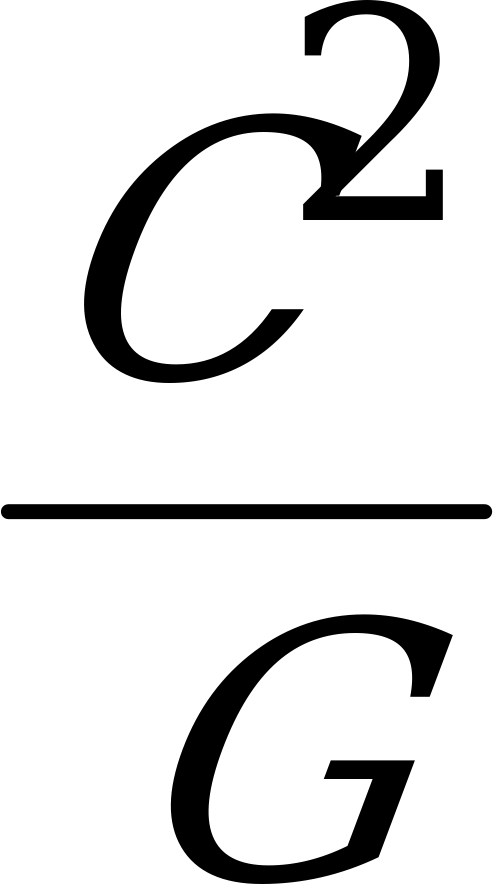, where: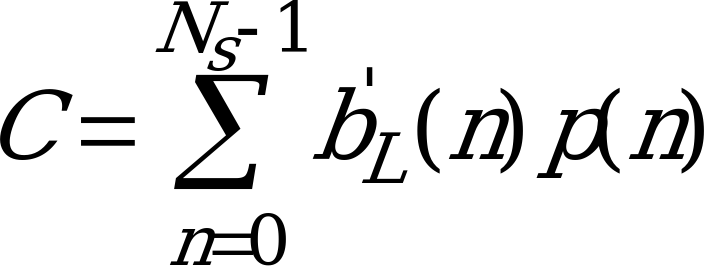(102)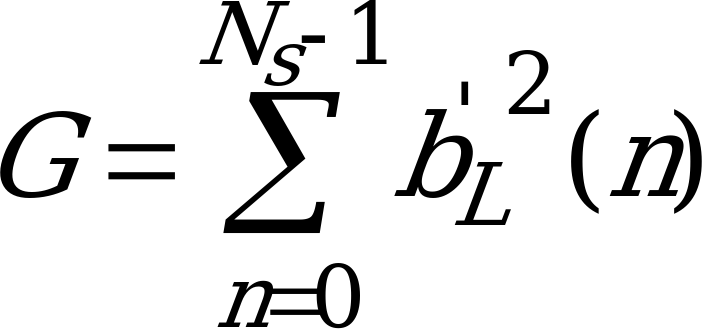(103)

The sequence b’L(n) is the zero state response of H(z) to the adaptive codebook output for lag L. The sequence p(n) is the input speech, weighted by the filter W(z), minus the zero input response of H(z). The error minimization is done over only those lags in the list supplied by the open loop search. The lag L which maximizes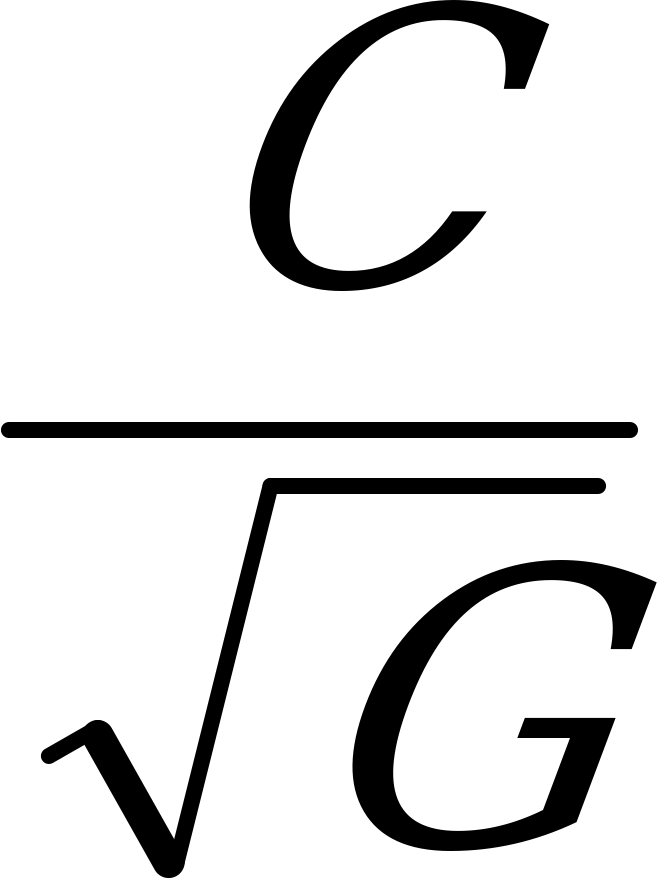(C is allowed to be negative) is then chosen as the lag for the subframe.